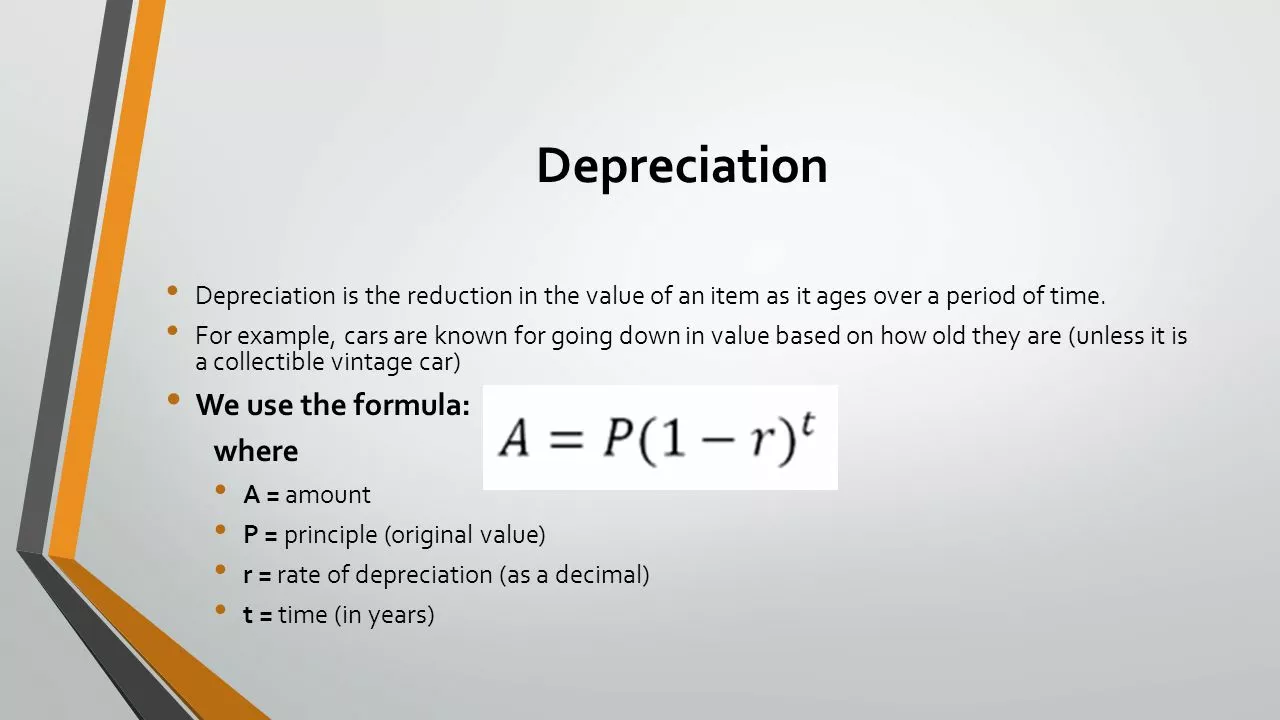# What is depreciation, types of depreciation?Miles Donovan## Understanding the Concept of Depreciation

Depreciation is a term that you've probably heard a lot in relation to business, finance, and economics. It's a critical component in the world of accounting and plays a significant role in determining the financial health of a business. But what exactly is depreciation? Simply put, depreciation is the reduction in the value of an asset over time, due to elements such as wear and tear, age, or obsolescence. Think of it like the aging of a car. As the car gets older, it's not as valuable as it used to be, and it's considered to be depreciating.

## The Importance of Accounting for Depreciation

Depreciation is a fundamental concept in accounting. It's crucial to take depreciation into account because it has a direct impact on a company's financial statements. By failing to account for depreciation, a business may overstate its assets and understate its expenses, leading to an inaccurate picture of the company's financial health. It’s like ignoring the fact that your car is getting older and needs repairs. Eventually, the costs will catch up with you. By accounting for depreciation, a business can ensure that its financial statements accurately reflect its financial position.

## How Depreciation is Calculated

Calculating depreciation can be a complex process, but generally, it involves determining the cost of the asset, its expected useful life, and its estimated residual value. Once these factors are determined, various methods can be employed to calculate the amount of depreciation each year. Some methods allocate the cost evenly over the asset's life, while others may allocate more cost to the early years of the asset's life. The method chosen can significantly affect the company's financial statements and tax liabilities.

## Straight-Line Depreciation Method

The straight-line depreciation method is the most commonly used. It's simple, straightforward, and easy to understand. Under this method, an equal amount of depreciation is charged each year over the useful life of the asset. For example, if a machine costs \$10,000, has a useful life of 10 years, and is expected to have a residual value of \$1,000, then the annual depreciation would be \$900 ((\$10,000 - \$1,000) / 10).

## Declining Balance Depreciation Method

The declining balance depreciation method is another common method. It's a bit more complicated than the straight-line method, but it can provide a more accurate representation of an asset's value over time. Under this method, a higher amount of depreciation is charged in the early years of the asset's life, with the amount decreasing each year. This method reflects the fact that assets often lose value more quickly in their early years.

## Sum-of-the-Years'-Digits Depreciation Method

The sum-of-the-years'-digits depreciation method is a bit more complex, but it can be a good option in certain situations. Like the declining balance method, it results in more depreciation in the early years of an asset's life. However, instead of a constant rate, the amount of depreciation decreases each year. This method can be a good option for assets that rapidly lose value early on but then level off.

## Units-of-Production Depreciation Method

The units-of-production depreciation method is a bit different from the other methods. Instead of basing depreciation on time, it bases it on the amount of use or production. For example, a machine might be depreciated based on the number of units it produces or the number of hours it's in use. This method can be a good option for assets whose value is more directly tied to how much they're used rather than how old they are.